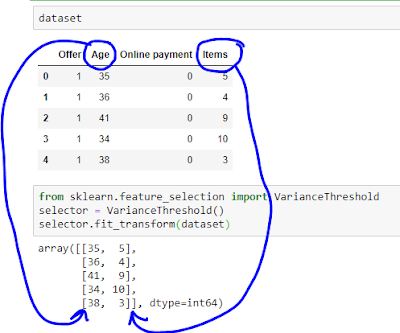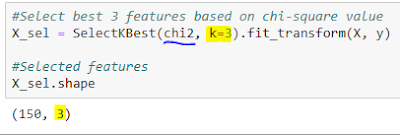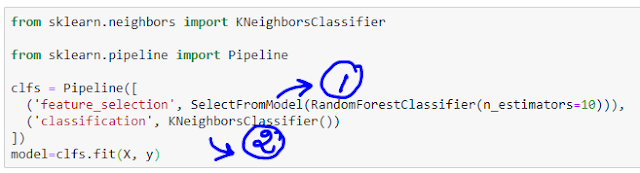### Feature Selection using sklearn

In this post, we will understand how to perform Feature Selection using sklearn.

• Dropping features which have low variance
• Dropping features with zero variance
• Dropping features with variance below the threshold variance
• Univariate feature selection
• Model based feature selection
• Feature Selection using pipeline
Let us start to explore these concepts one by one.

### 1) Dropping features which have low variance

If any features have low variance, they may not contribute in the model. For example, in the following dataset, features "Offer" and "Online payment" have zero variance, that means all the values are same. These two features can be dropped without any negative impact on the model to be built.

• A) Dropping features with zero variance

If a feature has same values across all observations, then we can remove that variable. In the following example, two features can be removed.Dataset with two features having zero variance

By default, variance threshold is zero in VarianceThreshold option in sklearn.feature_selection.Default variance threshold is zero

Using the following code, we can retain only the variables with non-zero variance.VarianceThreshold option drops two features with zero variance

• B) Dropping features with variance below the threshold variance
In the following example, dataset contains five features, out of which two features, "Referred" and "Repeat" do not vary much. Since data which contains values 0 and 1 are Bernoulli random variables, variance is given by the formula: p(1-p).Dataset with two features (Referred and Repeat) having low variance

If we want to retain a feature which contains only 0s 80% of the time or only 1s 80% of the time, then the variance of that feature would be: 0.8*(1-0.8)= 0.16.

We can mention VarianceThreshold(threshold=(.8 * (1 - .8))) or VarianceThreshold(threshold=0.16).Feature with either only 1s or 0s in 80% of the time is dropped

### 2) Univariate feature selection

In this type of selection method, a score is computed to capture the importance of feature. Score can be calculated using different measures such as Chi-square, F value, mutual information etc.

The following are the some of the options available in univariate feature selection.

Let us use the example provided by sklearn to understand how univariate feature selection works.

In the following example, original iris dataset contains four predictors.Original dataset contains four predictors

We want to retain only three predictors based on chi-square value.  The following code selects the top three features.Best three predictors are retained based on chi-square value

Scores used for regression problems are f_regression and mutual_info_regression.

In classification problems, chi-square value, f_classif and mutual_info_classif are the scores used.

### 3) Model based feature selection

• Recursive elimination
• L1-based selection
• Tree-based selection
In the following example, let us select best features in iris dataset using a model. Let us use random forest model to estimate feature importance.

Original dataset has four predictors.Using Random Forest model to select features

We can get the feature importance from the random forest classifier.Estimating feature importance

Using the feature importance, SelectFromModel option has retained only two features.Out of four, two features have been retained

You can see both the original dataset and the feature selected dataset below.Last two features have been retained

### 4) Feature Selection using pipeline

Using pipeline option, we can club the steps to select the features (step 1) and then use the selected features in the model training (step 2).Pipeline process: first features are selected and then using selected features model is built

### Summary

In this post, we have explored:
• Dropping features which have low variance
• Univariate feature selection
• Model based feature selection
• Feature Selection using pipeline
Which feature selection method you use often? Do share your views below.

If you liked my video, consider subscribing: